# Associators with Frozen Feet

Jump to: navigation, search

## The Goal

The purpose of the paperlet is to find an explicit formula for an associator with frozen feet. As I'm starting to write, I don't know such a formula. My hope is that as I type up all the relevant equations, a solution will emerge. I'll be just as happy if it emerges in somebody else's mind, provided (s)he shares her/his thoughts.

A horizontal associator is a solution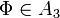$\Phi\in A_3$ of the pentagon equation and the hexagon equations (, , ):

 [Pentagon]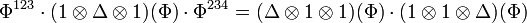$\Phi^{123}\cdot(1\otimes\Delta\otimes 1)(\Phi)\cdot\Phi^{234}=(\Delta\otimes 1\otimes 1)(\Phi)\cdot(1\otimes 1\otimes\Delta)(\Phi)$     in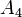$A_4$,

 [Hexagons]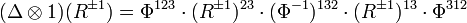$(\Delta\otimes 1)(R^{\pm 1}) = \Phi^{123}\cdot (R^{\pm 1})^{23}\cdot(\Phi^{-1})^{132}\cdot(R^{\pm 1})^{13}\cdot\Phi^{312}$     in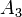$A_3$.

Here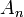$A_n$ is the algebra of horizontal chord diagrams on$n$ vertical strands; i.e.,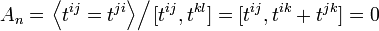$A_n=\left.\left\langle t^{ij}=t^{ji}\right\rangle\right/[t^{ij},t^{kl}]=[t^{ij},t^{ik}+t^{jk}]=0$, with$i$,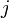$j$,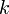$k$ and$l$ all different integers between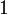$1$ and$n$. Also, here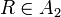$R\in A_2$ will be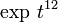$\exp\,t^{12}$ (slightly different than the normal convention of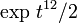$\exp\,t^{12}/2$, just to save some denominators).

The frozen feet quotient of any associative algebra$A$ is the quotient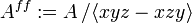$A^{ff}:=A\left/\left\langle xyz-xzy\right\rangle\right.$. (In a vertical presentation of chord diagrams we may think of the first letter of a word as its foot. The frozen feet relation makes all letters of a word commute, except perhaps the first one. "Molten bodies" is perhaps more accurate, but it is definitely less catchy).

What we are looking for is an associator with frozen feet, a solution of exactly the same equations, except regarded with the frozen feet quotients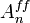$A^{ff}_n$ of$A_n$.

## Why Bother?

This isn't the place to explain the need for an explicit associator. The need is there even if it is a bit under-appreciated. Why frozen feet? Because finding an honest explicit horizontal associator seems too hard, and as a warm up and perhaps a step, it seems worthwhile to look for associators in some quotient spaces. The frozen feet quotient is the simplest quotient I'm aware off for which the answer is unknown.

## Why might it be doable?

Because frozen feet are really molten bodies. It is known that there exists and associator of the form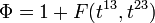$\Phi=1+F(t^{13},t^{23})$, where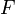$F$ is some non-commutative power series with no constant term. Once the body melts (but keeping the feet frozen), this becomes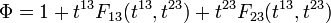$\Phi=1+t^{13}F_{13}(t^{13},t^{23})+t^{23}F_{23}(t^{13},t^{23})$,

where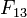$F_{13}$ and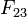$F_{23}$ are commutative power series (i.e., "functions"). So finding an associator with frozen feet reduces to finding two, just two, functions of two, just two, variables, satisfying some algebraic equations. How hard can that be?

Well, it's actually even easier. Taking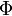$\Phi$ to be the projection from$A_3$ to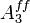$A^{ff}_3$ of a group like horizontal associator we find (by means to be documented later) that me may actually take it to be of the form

 [Phi]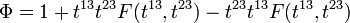$\Phi=1+t^{13}t^{23}F(t^{13},t^{23})-t^{23}t^{13}F(t^{13},t^{23})$,

where$F$ is a single unknown function of two commuting variables.

## So is it doable?

Me? Why are you asking me? My forte is in daydreaming; not in solving math problems. Anyway, let's try. The hexagon equations are easier (they are written in$A_3$ and not the "bigger"$A_4$) and in some sense more important (they force$\Phi$ to be non-trivial;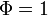$\Phi=1$ solves the pentagon but not the hexagons), so we will start with the hexagons. First we simply the hexagon in$A^{ff}_3$, factor by factor:

 Factor Written in$A^{ff}_3$ Comments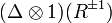$(\Delta\otimes 1)(R^{\pm 1})$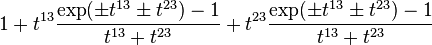$1+t^{13}\frac{\exp(\pm t^{13}\pm t^{23})-1}{t^{13}+t^{23}}+t^{23}\frac{\exp(\pm t^{13}\pm t^{23})-1}{t^{13}+t^{23}}$ We use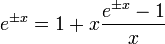$e^{\pm x}=1+x\frac{e^{\pm x}-1}{x}$ to write exponentials with their frozen parts made explicit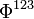$\Phi^{123}$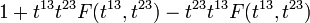$1+t^{13}t^{23}F(t^{13}, t^{23})-t^{23}t^{13}F(t^{13}, t^{23})$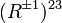$(R^{\pm 1})^{23}$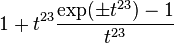$1+t^{23}\frac{\exp(\pm t^{23})-1}{t^{23}}$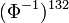$(\Phi^{-1})^{132}$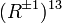$(R^{\pm 1})^{13}$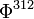$\Phi^{312}$